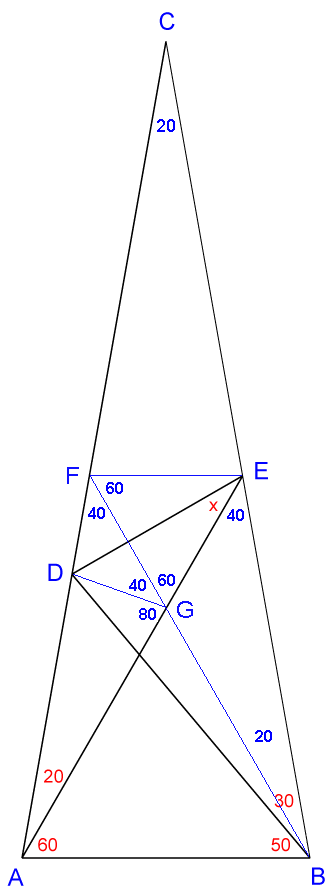# How to find measurements of angle CBD?I got $x + CBD = 110^{o}$. I think I should find measurements of angle CBD, so how to find it? Do you have any ideas? Please help me!

• The answer is $30^\circ$. – Tunk-Fey May 28 '14 at 17:21
• How to find x ? – Jung Hyun Ran May 28 '14 at 17:261. $\angle ACB = 180^\circ-(10^\circ+70^\circ)-(60^\circ+20^\circ) = 20^\circ$ and $\angle AEB = 180^\circ-60^\circ-(50^\circ+30^\circ) = 40^\circ$.
2. Draw a line from point $E$ parallel to $AB$, labeling the intersection with $AC$ as a new point $F$ and conclude $\Delta CEF\sim\Delta ABC$, \begin{align} \angle CEF &= \angle CBA = 50^\circ+30^\circ = 80^\circ\\ \angle FEB &= 180^\circ-80^\circ = 100^\circ\\ \angle AEF &= 100^\circ-40^\circ = 60^\circ\\ \angle CFE &= CAB = 60^\circ+20^\circ = 80^\circ\\ \angle EFA &= 180^\circ-80^\circ = 100^\circ \end{align}
3. Draw a line $FB$ labeling the intersection with $AE$ as a new point $G$ and conclude $\Delta AFE\cong\Delta BEF$, \begin{align} \angle AFB &=\angle BEA = 40^\circ\\ \angle BFE &= \angle AEF = 60^\circ\\ \angle FGE &= 180^\circ-60^\circ-60^\circ = 60^\circ = \angle AGB\\ \angle ABG &= 180^\circ-60^\circ-60^\circ = 60^\circ\\ \end{align}
4. Draw a line $DG$. Since $AD=AB$ (leg of isosceles) and $AG=AB$ (leg of equilateral), conclude $AD = AG$, $\Delta DAG$ is isosceles and $$\angle ADG =\angle AGD = \frac{180^\circ-20^\circ}2 = 80^\circ.$$
5. Since $\angle DGF = 180^\circ-80^\circ-60^\circ = 40^\circ$, conclude $\Delta FDG$ (with two $40^\circ$ angles) is isosceles, so $DF = DG$.
6. With $EF = EG$ (legs of equilateral) and $DE = DE$ (same line segment) conclude $\Delta DEF\cong\Delta DEG$ by side-side-side rule, $\angle DEF =\angle DEG = x$, and $$\angle FEG = 60^\circ = x+x\quad\Rightarrow\quad \large\color{blue}{x=30^\circ}.$$
• Did you mean $x=30^\circ$ at the end? – S.B. May 28 '14 at 18:04
• @S.B. Thanks for the correction. I fix the typo. – Tunk-Fey May 28 '14 at 18:11
• After the construction of FE and point G, CG is then the axis of symmetry. Thus, some of the angles can be found directly. – Mick May 29 '14 at 1:16
• Footnote - this interesting question also permits a solution by Sine Rule when using the 3 Isosceles triangles available, although it is no less complex than the solution given. – AlphaFSC May 29 '14 at 9:07
• @AlphaFSC Footnote, using trigonometry maybe will be the fastest way but I prefer using pure geometry approach in this case. – Tunk-Fey May 29 '14 at 9:12